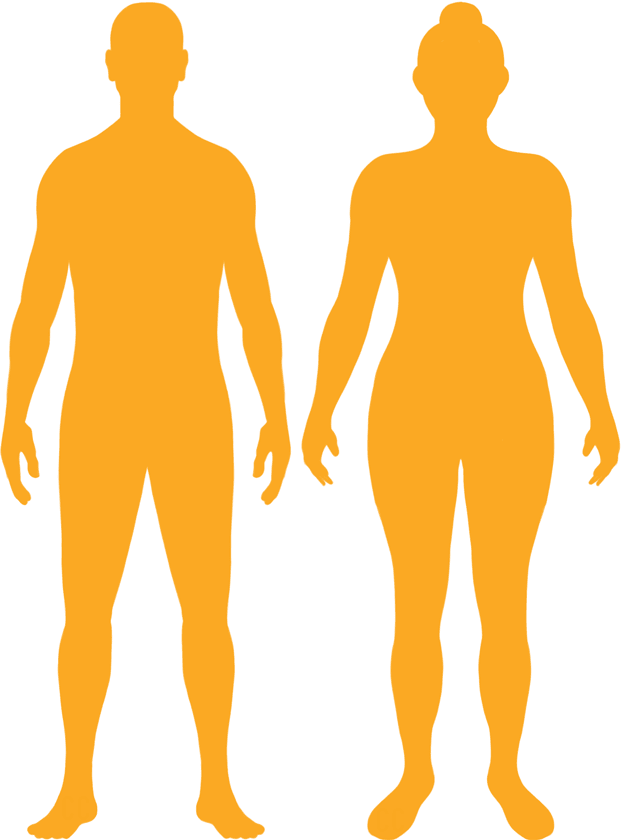# BMI Calculator

Result for 7'9" and 330 lbs.
Change Here.26.82

### Overweight BMI

At 7'9" and 330 Pounds your BMI is 26.82

### BMI Scale

 Under Normal Over Obese
-23 lbs
to reach a
normal BMI classification.
##### Normal BMI Ends:
307.5 lbs
+39 lbs
until you reach an obese BMI classification.
369.1 lbs

### BMI Grading Table2 for Height 7'9"

 Weight Weight Class Below 227.6 lbs Underweight 227.6 lbs - 307.5 lbs Normal Weight 307.5 lbs - 369.1 lbs Overweight 369.1 lbs and Above Obese

### Weight Loss

If you were seeking a normal BMI weight of 307.5lbs, and you lost two pounds a week4, then you could reach a normal BMI in
11.5 Weeks

### Overweight BMI Silhouette### Ideal Weight for a 7 foot 9 Male or Female2

The ideal weight range using the BMI for a male or female with a height of 7'9" is

### Between

227.6 lbs

and
307.5 lbs
How to Calculate BMI3 for 7'9" and 330 lbs
(7 x 12) + 9 = 93
(330 / (93 x 93)) x 703
= 26.822754075616
7 = foot | 9 = inch | 330 = weight lbs

### Conversion

• 7 foot 9 = 93 inches
• 7 foot 9 = 236.22cm
• 330 pounds = 23 stone 8 pounds
• 330 pounds = 149.69 kg
• 330 pounds = 149685.48 grams

### 7'9" and 330 lbs Summary

What is the BMI for a 7'9" and 330 lbs female? 26.82 BMI, Overweight.
What is the BMI for a 7'9" and 330 lbs male? 26.82 BMI, Overweight.
What is the ideal weight for a 7'9" female? Between: 227.6lbs and 307.5lbs
What is the ideal weight for a 7'9" male? Between: 227.6lbs and 307.5lbs
If I am 7ft 9in and weigh 330 lbs, is that a good weight for my height? Under the BMI classification, 330 lbs is classed as being Overweight.